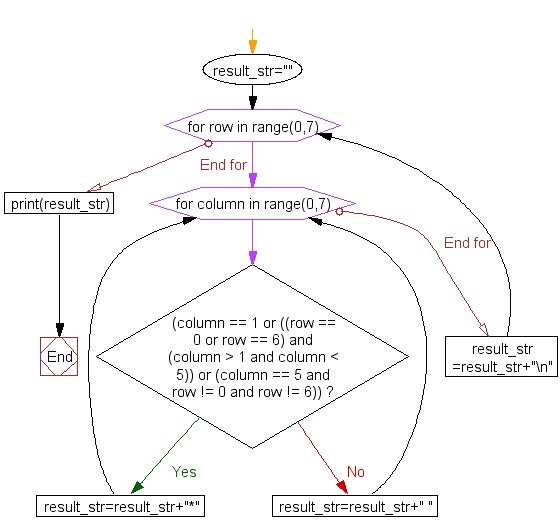﻿ Python Exercise: Print alphabet pattern D - w3resource

# Python Exercise: Print alphabet pattern D

## Python Conditional: Exercise - 18 with Solution

Write a Python program to print the alphabet pattern 'D'.

Pictorial Presentation:Sample Solution:

Python Code:

``````# Initialize an empty string named 'result_str'
result_str = ""

# Iterate through rows from 0 to 6 using the range function
for row in range(0, 7):
# Iterate through columns from 0 to 6 using the range function
for column in range(0, 7):
# Check conditions to determine whether to place '*' or ' ' in the result string

# If conditions are met, place '*' in specific positions based on row and column values
if (column == 1 or ((row == 0 or row == 6) and (column > 1 and column < 5)) or (column == 5 and row != 0 and row != 6)):
result_str = result_str + "*"  # Append '*' to the 'result_str'
else:
result_str = result_str + " "  # Append space (' ') to the 'result_str'

result_str = result_str + "\n"  # Add a newline character after each row in 'result_str'

# Print the final 'result_str' containing the pattern
print(result_str)
```
```

Sample Output:

``` ****
*   *
*   *
*   *
*   *
*   *
****  ```

Flowchart :Python Code Editor:

Have another way to solve this solution? Contribute your code (and comments) through Disqus.

What is the difficulty level of this exercise?

Test your Programming skills with w3resource's quiz.

﻿